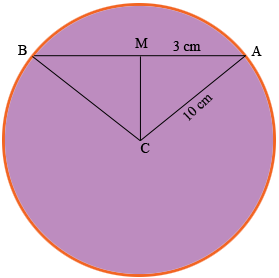SEARCH HOMEMath Central Quandaries & QueriesQuestion from Mujtaba, a student: The distance of a chord of length 16 cm, from the centre of the circle of diameter 20 cmHi Mujtaba,

In my diagram the chord is $AB$ and $M$ is the midpoint of $AB.$What do you know about angle $AMC?$ Why? What does Pythagoras tell you?

PennyMath Central is supported by the University of Regina and the Imperial Oil Foundation.